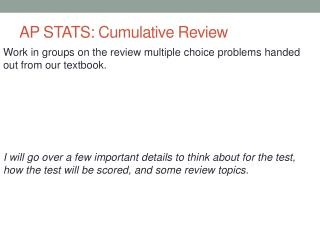DownloadDownload PresentationAP STATS: Cumulative Review

# AP STATS: Cumulative Review

Download Presentation## AP STATS: Cumulative Review

- - - - - - - - - - - - - - - - - - - - - - - - - - - E N D - - - - - - - - - - - - - - - - - - - - - - - - - - -
##### Presentation Transcript

1. AP STATS: Cumulative Review Work in groups on the review multiple choice problems handed out from our textbook. I will go over a few important details to think about for the test, how the test will be scored, and some review topics.

2. Answers • 1.) D • 2.) A • 3.) E • 4.) A • 5.) C • 6.) A • 7.) E • 8.) E • 9.) D • 10.) C

3. CUMULATIVE TEST TOPICS Chapter 1: Exploring Data Displaying Distributions with Graphs (stemplots, histograms, boxplots, ogives etc.) -Describing Distributions with Numbers (mean, median, standard deviation quartiles etc.) Chapter 2: Describing Location in a Distribution -Measures of relative standing and density curves -Normal Distributions (z-tables) Chapter 3: Examining Relationships Between 2 Variables -Scatter Plots -Linear Regressions(least squares) -Correlation (r) and Correlation of determination (r-squared). -Residual: y-hat = actual - predicted Chapter 4: More about Relationships between 2 Variables -Transforming to Achieve Linearity (x, log y) (log x, log y) etc. - Categorical Variables (two way tables, marginal distributions, -Establishing Causation (Correlation does NOT imply causation)

4. AP STATISTICS SCORING